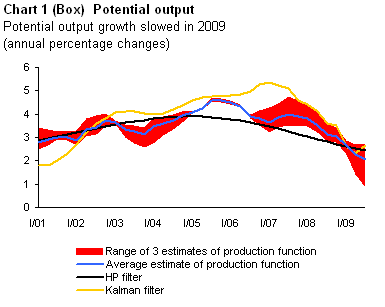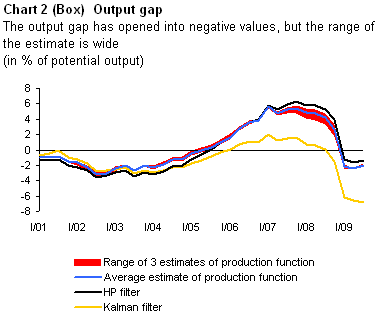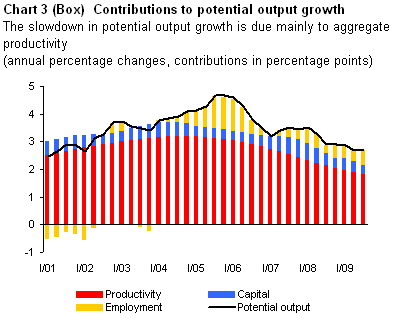# Uncertainties surrounding the calculation of potential output

In an environment of global crisis, the Czech economy saw a shift in the business cycle in 2008 and 2009. The current position in the business cycle is illustrated by the output gap, which is the percentage deviation of real output from potential output, expressing the equilibrium level of output. Like the equilibrium levels of other macroeconomic variables, however, potential output is not observable and measurable. There is thus considerable uncertainty about its level and growth rate, particularly in the present situation, and a whole range of different methods can be used to estimate it.

The first method currently used in the CNB to estimate potential output is based on the Cobb-Douglas production function (in its basic form), which represents the supply side of the economy and expresses the relationship between the volume of inputs (production factors – aggregate productivity, labour and capital) and output (product), with productivity being calculated and subsequently smoothed using the Hodrick-Prescott filter. The advantage of this potential output calculation method is the possibility of analysing the contributions of the individual factors (productivity, labour and capital) to potential output growth. In the CNB, the production function is computed in three variants using different input data.

The second method applied in the CNB to calculate potential output uses a multi-equation model estimated using a recursive algorithm known as the Kalman filter. The advantage of this model is that in addition to data concerning economic activity directly, as measured by GDP, it includes other information, for example regarding the labour market and inflation.

The third, and technically simplest, method used to estimate potential output involves application of the Hodrick-Prescott (HP) filter to the GDP time series. This univariate filter (i.e. using only one time series) is used quite often to filter the trend and cyclical parts of a time series. Its drawback consists in suffering from an end-point bias, particularly if the cycle changes phase. To mitigate this problem, the HP filter calculation is performed at the CNB on the time series including the forecast.All the above methods for calculating potential output are applied at the CNB to quarterly data from 1997. Their results show that the annual decline in GDP in 2009 was associated with a significant slowdown in the growth rate of potential output (see Chart 1). The Cobb-Douglas production function calculation indicates that the potential output growth rate decreased to 2.1% in 2009 Q3 (the average of the three variants), while the alternative estimates using the Kalman filter and HP filter provide slightly higher values.At the start of 2009, the corresponding output gap turned negative and stayed there throughout 2009 (see Chart 2). The Cobb-Douglas production function estimate indicates a not-too-wide opening of the output gap to negative values (-2% of potential output) following a previous significant overheating of the economy in 2006–2008, whereas the Kalman filter puts the current output gap almost 5 percentage points wider. The range of the individual results thus indicates a high degree of uncertainty.A look at the contributions of the individual production factors entering one of the variants of the Cobb-Douglas production function reveals that the slowdown in potential output growth is due to slowing growth in all input factors, but mainly in productivity (see Chart 3). The decline in employment has a lagged effect and its negative contributions to potential output growth can thus be expected to appear during 2010.